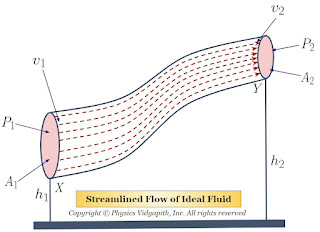## Origin of Fossil Fuels

Fossil fuels are formed over millions of years from the remains of plants and animals which died and were buried under sediment. The process of their formation involves several stages, which are described below:

Formation of Organic Matter: Fossil fuels are formed from organic matter, which includes the remains of plants, animals, and microorganisms. These organisms lived in ancient swamps, oceans, and forests, and their remains were buried under sediment over time.

Deposition and Burial: Once the organic matter is buried under sediment, it is protected from decomposition by the lack of oxygen. The sediment continues to accumulate on top of the organic matter, causing it to be buried deeper and deeper.

Pressure and Heat: As the organic matter is buried deeper, the weight of the overlying sediment increases, causing pressure to build up. The pressure and heat from the overlying sediment and rock cause the organic matter to undergo chemical and physical changes, leading to the formation of fossil fuels.

Formation of Coal: Coal is formed from the remains of plants that were buried under sediment and subjected to high pressure and temperature over millions of years. The process of coal formation involves the transformation of plant material into peat, lignite, and finally, coal.

Formation of Oil and Gas: Oil and natural gas are formed from the remains of tiny marine organisms, such as plankton, that lived in the oceans millions of years ago. When these organisms died, their remains settled on the ocean floor and were buried under sediment. Over time, the heat and pressure from the overlying sediment and rock caused the organic matter to be transformed into oil and natural gas.

Migration and Trapping: Once formed, oil and natural gas migrate through the surrounding rock until they become trapped in a reservoir. The reservoir is a porous rock formation that can hold oil and natural gas, and it is usually sealed by an impermeable layer of rock, such as shale.

Extraction: Fossil fuels are extracted from the ground using various methods, such as drilling or mining. The extracted fossil fuels are then transported to refineries, where they are processed into various products, such as gasoline, diesel, and jet fuel. Overall, the formation of fossil fuels is a slow and complex process that takes millions of years. As a non-renewable resource, fossil fuels are finite and will eventually be depleted, making it important for us to find and develop alternative sources of energy that are sustainable and environmentally friendly.

## Popular Posts

### Bernoulli's Theorem and Derivation of Bernoulli's EquationStatement of Bernoulli's Theorem: When an ideal fluid (i.e incompressible and non-viscous Liquid or Gas) flows in streamlined motion from one place to another, then the total energy per unit volume (i.e Pressure energy + Kinetic Energy + Potential Energy) at each and every of its path is constant. $P+\frac{1}{2}\rho v^{2} + \rho gh= constant$ Derivation of Bernoulli's Theorem Equation: Let us consider that an incompressible and non-viscous liquid is flowing in streamlined motion through a tube $XY$ of the non-uniform cross-section. Now Consider: The Area of cross-section $X$ = $A_{1}$ The Area of cross-section $Y$ = $A_{2}$ The velocity per second (i.e. equal to distance) of fluid at cross-section $X$ = $v_{1}$ The velocity per second (i.e. equal to distance) of fluid at cross-section $Y$ = $v_{2}$ The Pressure of fluid at cross-section $X$ = $P_{1}$ The Pressure of fluid at cross-section $Y$ = $P_{2}$ The height of cross-section $X$ from